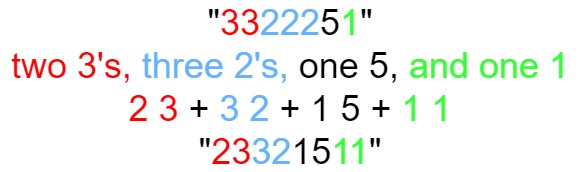# 外观数列

4 分钟读完

## 38. 外观数列 (Medium)

「外观数列」是一个整数序列，从数字 1 开始，序列中的每一项都是对前一项的描述。

• `countAndSay(1) = "1"`
• `countAndSay(n)` 是对 `countAndSay(n-1)` 的描述，然后转换成另一个数字字符串。

```1.     1
2.     11
3.     21
4.     1211
5.     111221

```描述前一项，这个数是 `11` 即 “ 二 个 1 ” ，记作 ```"21"
```描述前一项，这个数是 `21` 即 “ 一 个 2 + 一 个 1 ” ，记作 "```1211"
```描述前一项，这个数是 `1211` 即 “ 一 个 1 + 一 个 2 + 二 个 1 ” ，记作 "`111221"`
``````输入：n = 1

```

```输入：n = 4

countAndSay(1) = "1"
countAndSay(2) = 读 "1" = 一 个 1 = "11"
countAndSay(3) = 读 "11" = 二 个 1 = "21"
countAndSay(4) = 读 "21" = 一 个 2 + 一 个 1 = "12" + "11" = "1211"
```

• `1 <= n <= 30`

[字符串]

### 相似题目

1. 字符串的编码与解码 (Medium)
2. 压缩字符串 (Medium)